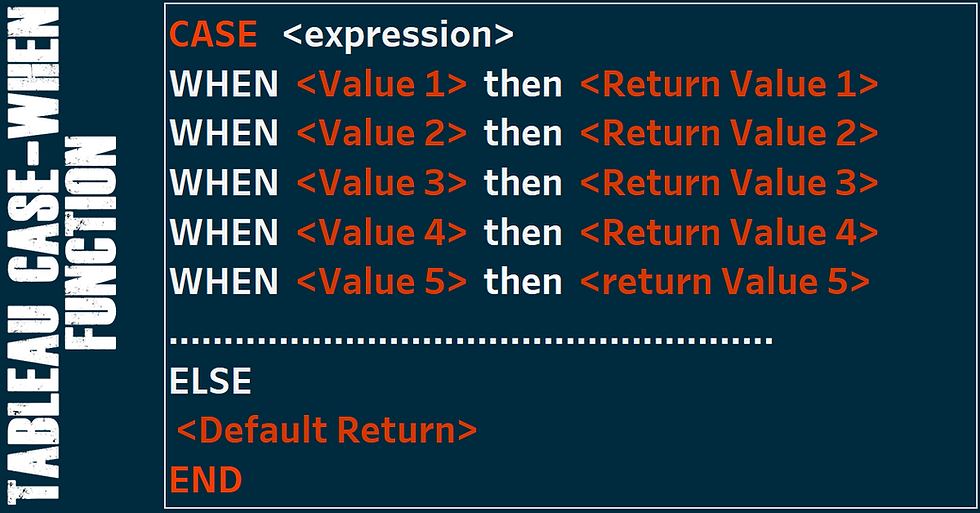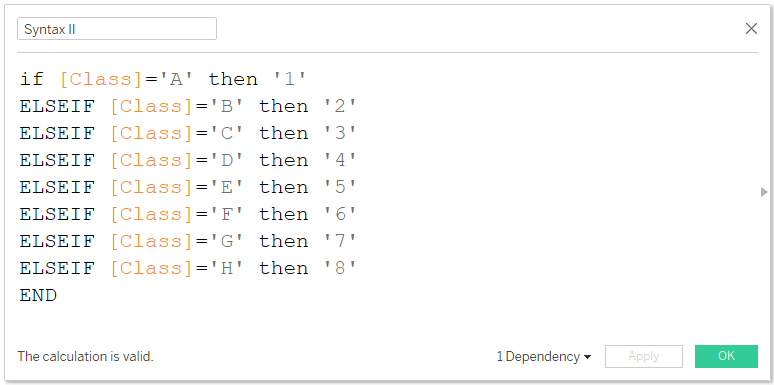top of page
Search

# Tableau Case Function

Updated: Nov 8, 2022Tableau CASE function applies the syntax (CASE-WHEN) in the following order to perform logical tests.The CASE function evaluates <expression> , compares it to a sequence of values, <Value 1>, <Value 2>, <Value 3>, <Value 4>, …etc, and returns a result. When a value that matches the expression is encountered, CASE returns the corresponding return value, <Return Value 1>, <Return Value 2>, <Return Value 3>, <Return Value 4>, …etc. If no match is found, then default return expression is used <Default Return>. If there is no default return expression and no values match, then Null is returned.

### Example of a CASE-WHEN statement

Suppose you have a data set with the field named ‘Class’ and the following as members of this field (‘A’, ’B’, ‘C’, ‘D’, ‘E’, ‘F’, ‘G’ , ‘H’, ’11’ & ‘20’). Let’s say, using CASE-WHEN function you would like to assign the alphabets as follows (‘1’ to ‘A’, ‘2’ to ‘B’……. ‘8’ to ‘H’) and any other number to ‘Remain as default’ or ‘Null’.

Then CASE-WHEN would be an ideal function to perform this, and when applied in the following two ways would return the following.

### Syntax I### Syntax IIWhen both the two syntaxes are applied in this data the result would be as per the table below;Note: The difference between the two syntaxes is, one defines what value to assign in case there is no match found while the other does not.

### Nested CASE-WHEN statement

Sometimes you can nest CASE-WHEN statement as follows to match values.

### Syntax IIIUsing the above syntax in this data would result to;### IF THEN ELSEIF statement

The above CASE-WHEN statements could also be replaced by an IF THEN ELSEIF statement as follows,

The first syntax ‘Syntax I’ could also be written.The second syntax ‘Syntax II’ could also be written.The third syntax ‘Syntax III’ could also be written.### Recommended Articles

I hope this article shed light on how to work with CASE -WHEN function.

To receive more of these Tableau tips and tricks, kindly subscribe to our mailing list below.

If you like the work we do and would like to work with us, drop us an email on our Contacts page and we’ll reach out!